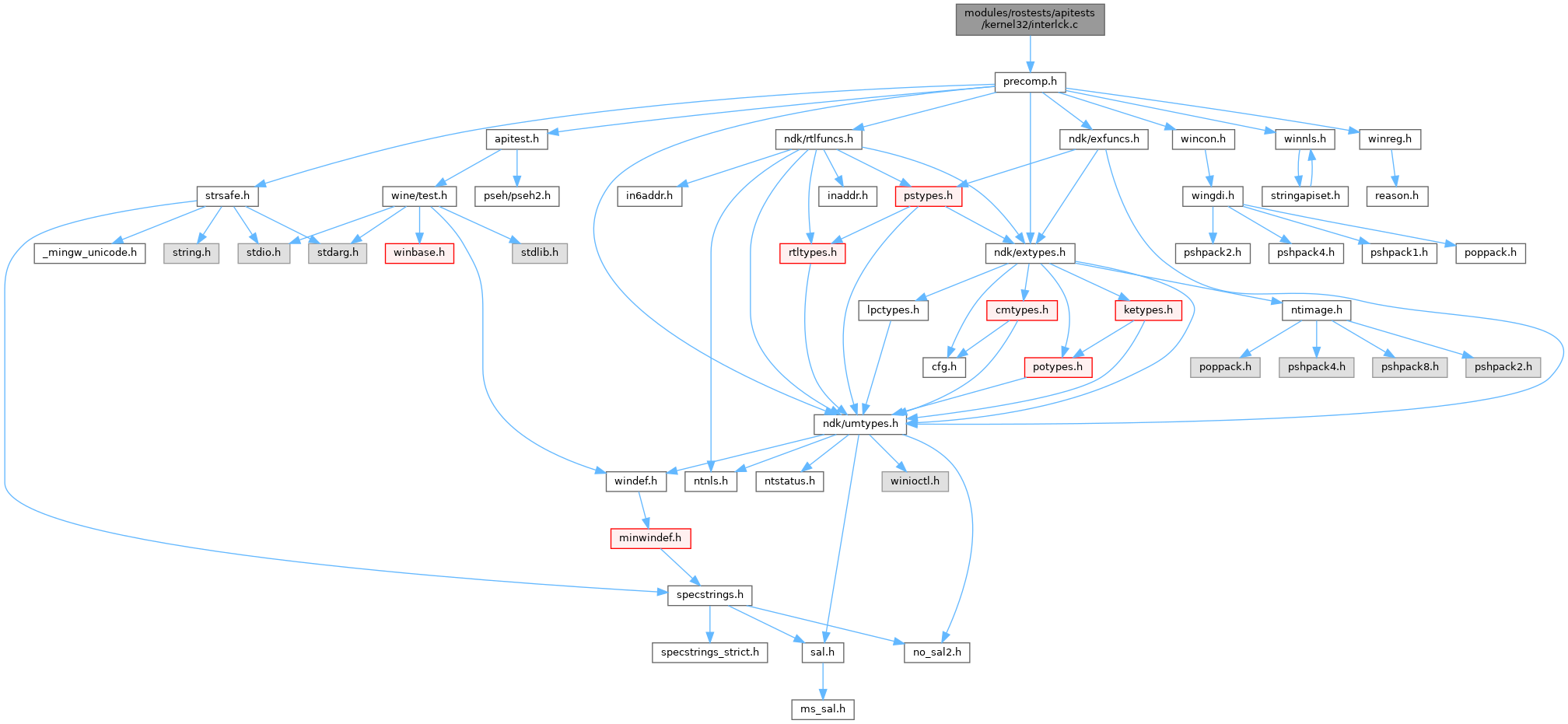ReactOS 0.4.15-dev-6067-g0b695a6
interlck.c File Reference
`#include "precomp.h"`
Include dependency graph for interlck.c:Go to the source code of this file.

## Functions

static void test_InterlockedCompareExchange (void)

static void test_InterlockedDecrement (void)

static void test_InterlockedExchange (void)

static void test_InterlockedIncrement (void)

START_TEST (interlck)

## ◆ START_TEST()

 START_TEST ( interlck )

Definition at line 118 of file interlck.c.

119{
125}
static void test_InterlockedIncrement(void)
Definition: interlck.c:98
static void test_InterlockedDecrement(void)
Definition: interlck.c:38
static void test_InterlockedExchange(void)
Definition: interlck.c:58
Definition: interlck.c:78
static void test_InterlockedCompareExchange(void)
Definition: interlck.c:23

## ◆ test_InterlockedCompareExchange()

 static void test_InterlockedCompareExchange ( void )
static

Definition at line 23 of file interlck.c.

24{
25 LONG dest, res;
26
27 dest = 0;
29 ok( res == 0 && dest == 1,
30 "Expected 0 and 1, got %ld and %ld", res, dest );
31
32 dest = 1;
34 ok( res == 1 && dest == 1,
35 "Expected 1 and 1, got %ld and %ld", res, dest );
36}
#define ok(value,...)
Definition: atltest.h:57
GLuint res
Definition: glext.h:9613
#define InterlockedCompareExchange
Definition: interlocked.h:104
static char * dest
Definition: rtl.c:135
long LONG
Definition: pedump.c:60

Referenced by START_TEST().

## ◆ test_InterlockedDecrement()

 static void test_InterlockedDecrement ( void )
static

Definition at line 38 of file interlck.c.

39{
40 LONG dest, res;
41
42 dest = 1;
44 ok( res == 0 && dest == 0,
45 "Expected 0 and 0, got %ld and %ld", res, dest );
46
47 dest = 0;
49 ok( res == -1 && dest == -1,
50 "Expected -1 and -1, got %ld and %ld", res, dest );
51
52 dest = -1;
54 ok( res == -2 && dest == -2,
55 "Expected -2 and -2, got %ld and %ld", res, dest );
56}
#define InterlockedDecrement
Definition: armddk.h:52

Referenced by START_TEST().

## ◆ test_InterlockedExchange()

 static void test_InterlockedExchange ( void )
static

Definition at line 58 of file interlck.c.

59{
60 LONG dest, res;
61
62 dest = 0;
64 ok( res == 0 && dest == 1,
65 "Expected 0 and 1, got %ld and %ld", res, dest );
66
67 dest = 1;
69 ok( res == 1 && dest == 2,
70 "Expected 1 and 2, got %ld and %ld", res, dest );
71
72 dest = 1;
74 ok( res == 1 && dest == -1,
75 "Expected 1 and -1, got %ld and %ld", res, dest );
76}
#define InterlockedExchange
Definition: armddk.h:54

Referenced by START_TEST().

 static void test_InterlockedExchangeAdd ( void )
static

Definition at line 78 of file interlck.c.

79{
80 LONG dest, res;
81
82 dest = 0;
84 ok( res == 0 && dest == 1,
85 "Expected 0 and 1, got %ld and %ld", res, dest );
86
87 dest = 1;
89 ok( res == 1 && dest == 3,
90 "Expected 1 and 3, got %ld and %ld", res, dest );
91
92 dest = 1;
94 ok( res == 1 && dest == 0,
95 "Expected 1 and 0, got %ld and %ld", res, dest );
96}
Definition: interlocked.h:181

Referenced by START_TEST().

## ◆ test_InterlockedIncrement()

 static void test_InterlockedIncrement ( void )
static

Definition at line 98 of file interlck.c.

99{
100 LONG dest, res;
101
102 dest = -2;
104 ok( res == -1 && dest == -1,
105 "Expected -1 and -1, got %ld and %ld", res, dest );
106
107 dest = -1;
109 ok( res == 0 && dest == 0,
110 "Expected 0 and 0, got %ld and %ld", res, dest );
111
112 dest = 0;
114 ok( res == 1 && dest == 1,
115 "Expected 1 and 1, got %ld and %ld", res, dest );
116}
#define InterlockedIncrement
Definition: armddk.h:53

Referenced by START_TEST().